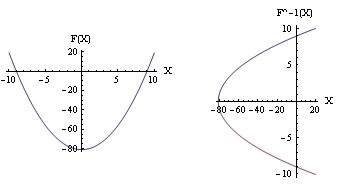# Let f(x) = x2 – 81. Find f–1(x)?

We can solve for x
f(x) = x^2 - 81
f(x) + 81 = x^2
√(f(x) + 81) = x